# Frames, ticks, titles, and labels

Setting frame, ticks, title, etc., of the plot is handled by the `frame` parameter that most plotting methods of the `pygmt.Figure` class contain.

```import pygmt
```

## Plot frame

By default, PyGMT does not add a frame to your plot. For example, we can plot the coastlines of the world with a Mercator projection:

```fig = pygmt.Figure()
fig.coast(shorelines="1/0.5p", region=[-180, 180, -60, 60], projection="M25c")
fig.show()
```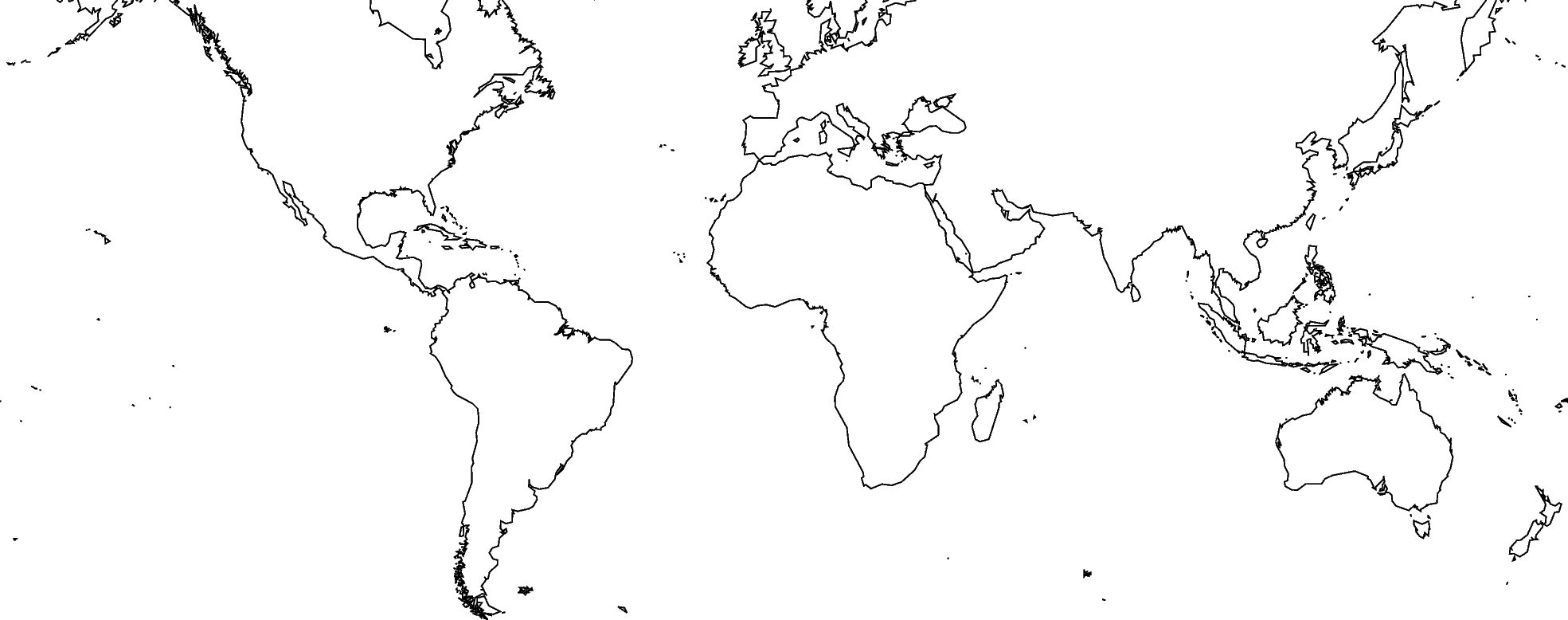To add the default GMT frame style to the plot, use `frame="f"` in `pygmt.Figure.basemap` or another plotting method (which has the `frame` parameter, with exception of `pygmt.Figure.colorbar`):

```fig = pygmt.Figure()
fig.coast(shorelines="1/0.5p", region=[-180, 180, -60, 60], projection="M25c")
fig.basemap(frame="f")
fig.show()
```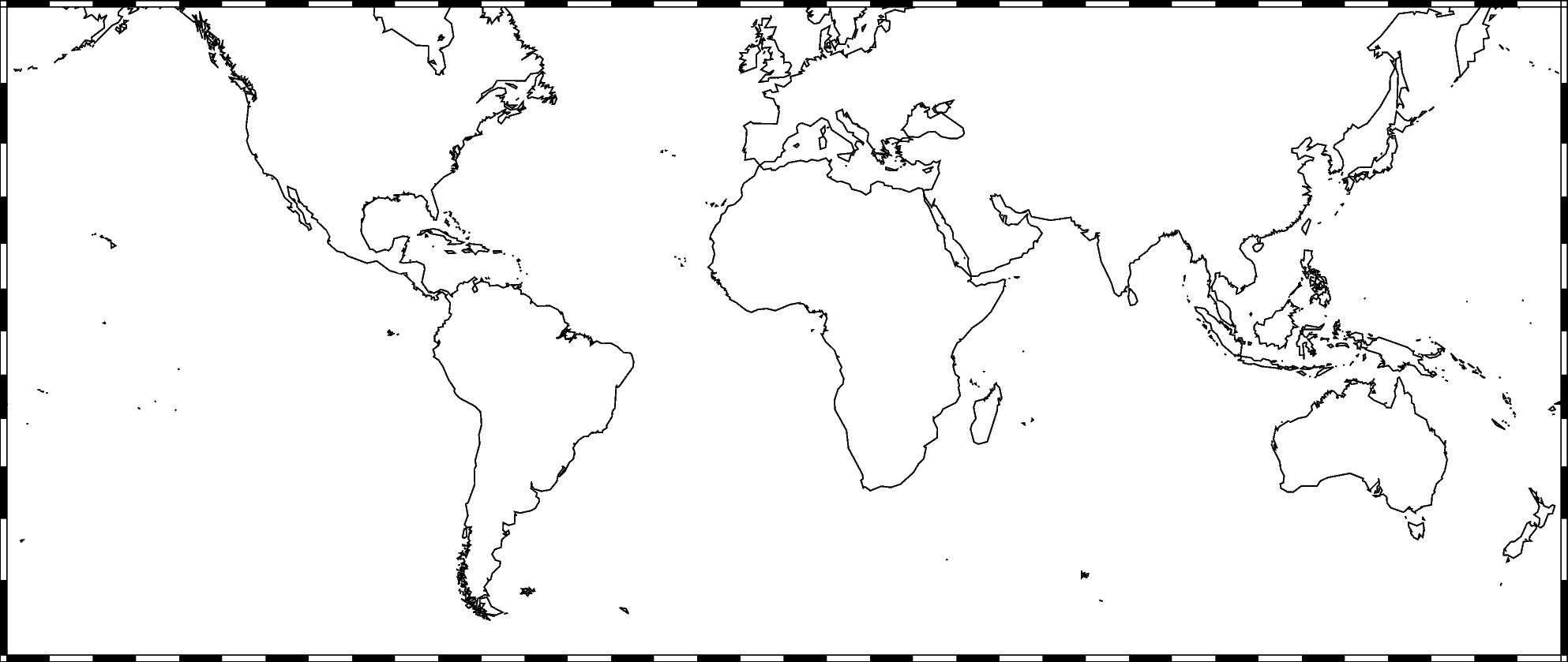## Ticks and grid lines

The automatic frame (`frame=True` or `frame="af"`) adds the default GMT frame style and automatically determines tick labels from the plot region. In GMT the tick labels are called annotations.

```fig = pygmt.Figure()
fig.coast(shorelines="1/0.5p", region=[-180, 180, -60, 60], projection="M25c")
fig.basemap(frame="af")
fig.show()
```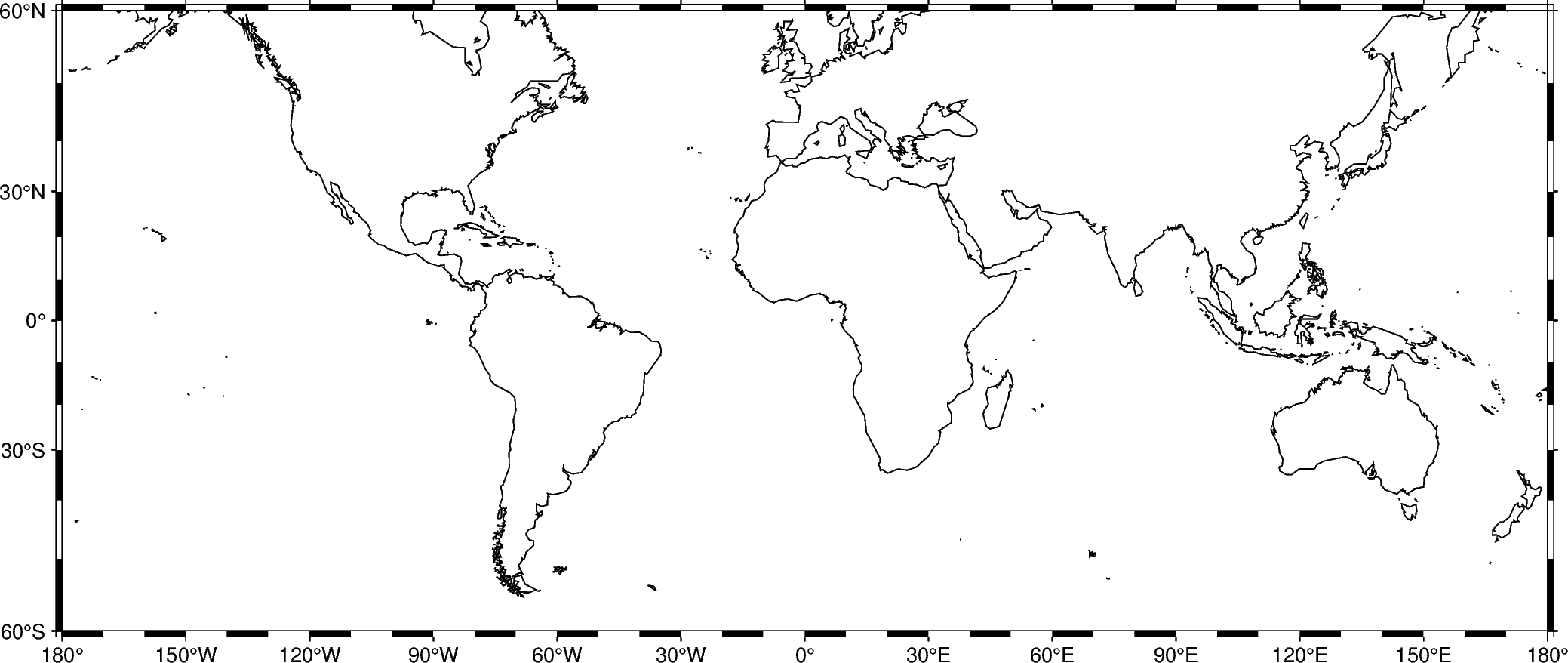Add automatic grid lines to the plot by passing `g` through the `frame` parameter:

```fig = pygmt.Figure()
fig.coast(shorelines="1/0.5p", region=[-180, 180, -60, 60], projection="M25c")
fig.basemap(frame="ag")
fig.show()
```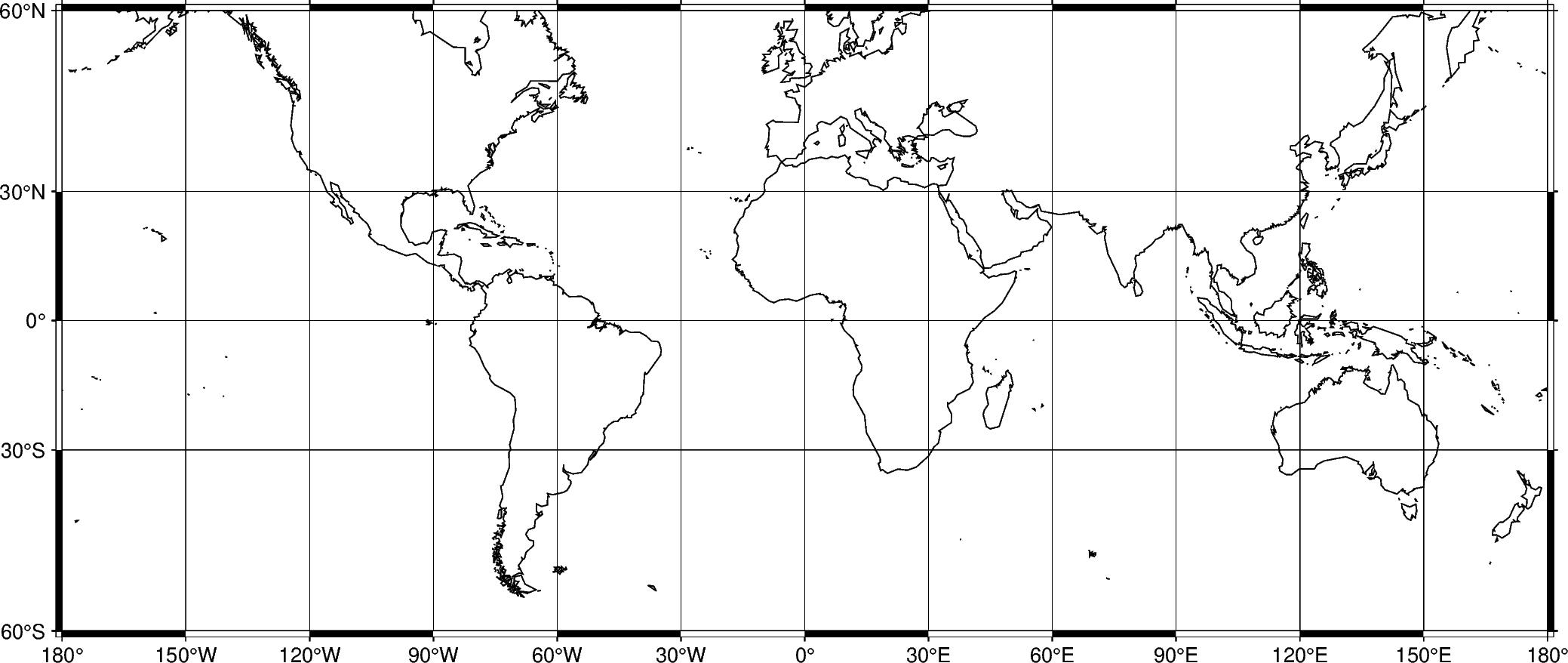To adjust the step widths of annotations, frame, and grid lines we can add the desired step widths after `a`, `f`, or `g`. In the example below, the step widths are set to 30°, 7.5°, and 15°, respectively.

```fig = pygmt.Figure()
fig.coast(shorelines="1/0.5p", region=[-180, 180, -60, 60], projection="M25c")
fig.basemap(frame="a30f7.5g15")
fig.show()
```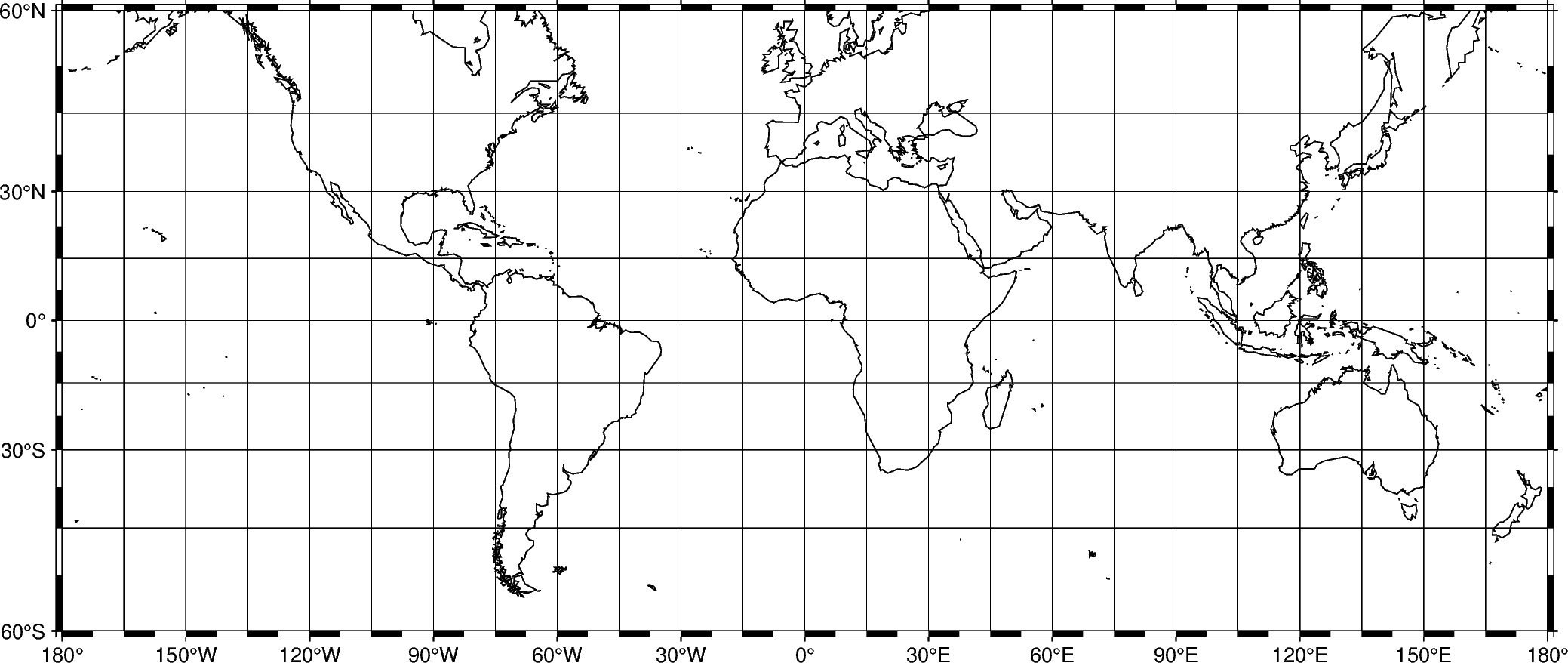## Title

The figure title can be set by passing +ttitle to the `frame` parameter of `pygmt.Figure.basemap`. Passing multiple arguments to `frame` can be done by using a list, as show in the example below.

```fig = pygmt.Figure()
# region="TT" specifies Trinidad and Tobago using the ISO country code
fig.coast(shorelines="1/0.5p", region="TT", projection="M25c")
fig.show()
```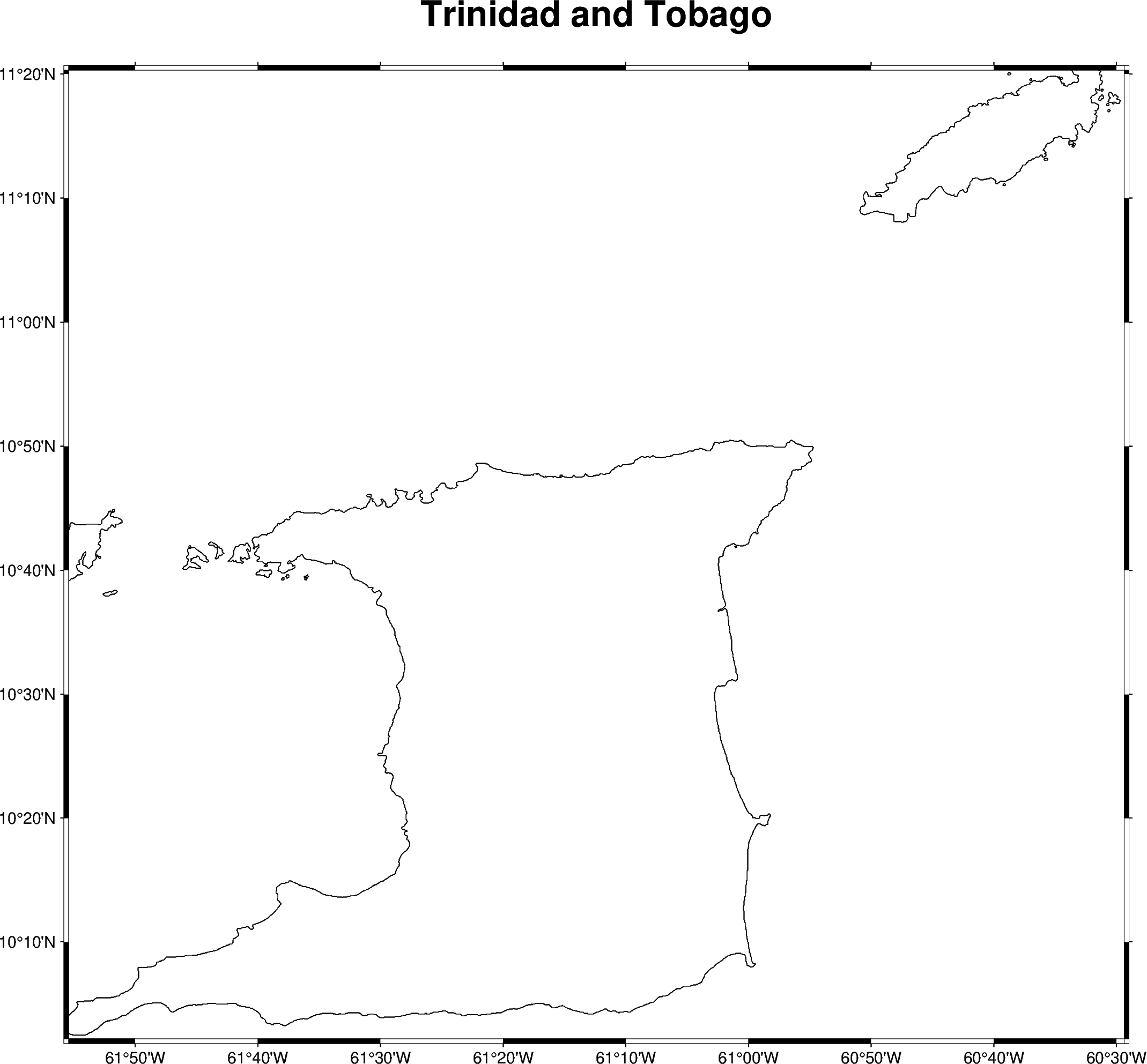## Axis labels

Axis labels, in GMT simply called labels, can be set by passing x+llabel (or starting with y if labeling the y-axis) to the `frame` parameter of `pygmt.Figure.basemap`. The map boundaries (or plot axes) are named as West/west/left (W, w, l), South/south/bottom (S, s, b), North/north/top (N, n, t), and East/east/right (E, e, r) sides of a figure. If an upper-case letter (W, S, N, E) is passed, the axis is plotted with tick marks and annotations. The lower-case version (w, s, n, e) plots the axis only with tick marks. To only plot the axis pass l, b, t, r. By default (`frame=True` or `frame="af"`), the West and the South axes are plotted with both tick marks and annotations.

The example below uses a Cartesian projection, as GMT does not allow labels to be set for geographic maps.

```fig = pygmt.Figure()
fig.basemap(
region=[0, 10, 0, 20],
projection="X10c/8c",
# Plot axis with tick marks, annotations, and labels on the
# West and South axes
# Plot axis with tick marks on the north and east axes
frame=["WSne", "xaf+lx-axis", "yaf+ly-axis"],
)
fig.show()
```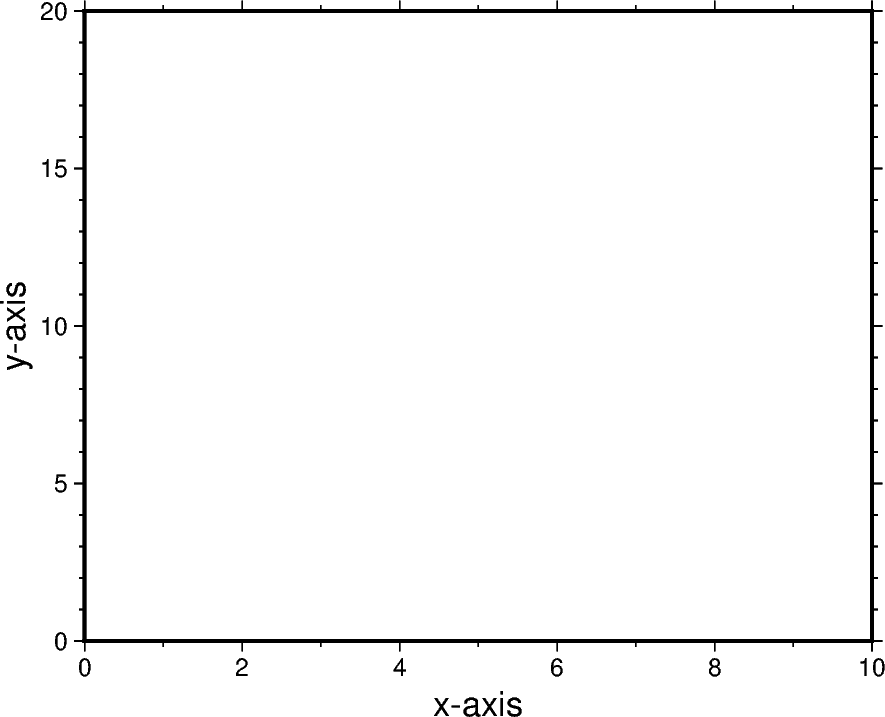Total running time of the script: ( 0 minutes 5.043 seconds)

Gallery generated by Sphinx-Gallery